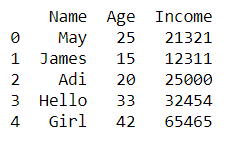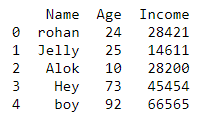Open in App
Not now

# How to remove numbers from string in Python – Pandas?

• Difficulty Level : Expert
• Last Updated : 25 Feb, 2021

In this article, let’s see how to remove numbers from string in Pandas. Currently, we will be using only the .csv file for demonstration purposes, but the process is the same for other types of files. The function read_csv() is used to read CSV files.

Syntax:

for the method ‘replace()’:

str.replace(old, new)

Here str. replace() will return a string in which the parameter ‘old’ will be replaced by the parameter ‘new’. Now let us see through coding how to remove numbers from strings in the pandas data frame.

Example 1:

## Python3

 `# code``import` `pandas as pd`` ` ` ` `# creating dataframe``df ``=` `pd.DataFrame.from_dict({``'Name'``: [``'May21'``, ``'James'``,``                                      ``'Adi22'``, ``'Hello'``,``                                      ``'Girl90'``],``                              ` `                             ``'Age'``: [``25``, ``15``, ``20``, ``33``, ``42``],``                              ` `                              ` `                             ``'Income'``: [``21321``, ``12311``, ``25000``,``                                        ``32454``, ``65465``]})`` ` `# removing numbers from strings of speciafied ``# column, here 'Name'``df[``'Name'``] ``=` `df[``'Name'``].``str``.replace(``'\d+'``, '')`` ` `# display output with numbers removed from ``# required strings``print``(df)`

Output:Example 2:

## Python3

 `# code``import` `pandas as pd`` ` ` ` `# creating dataframe``df ``=` `pd.DataFrame.from_dict({``'Name'``: [``'rohan21'``, ``'Jelly'``,``                                      ``'Alok22'``, ``'Hey65'``,``                                      ``'boy92'``],``                              ` `                             ``'Age'``: [``24``, ``25``, ``10``, ``73``, ``92``],``                              ` `                             ``'Income'``: [``28421``, ``14611``, ``28200``,``                                        ``45454``, ``66565``]})`` ` `# removing numbers from strings of speciafied ``# column, here 'Name'``df[``'Name'``] ``=` `df[``'Name'``].``str``.replace(``'\d+'``, '')`` ` `# display output with numbers removed from ``# required strings``print``(df)`

Output:My Personal Notes arrow_drop_up